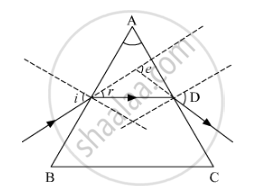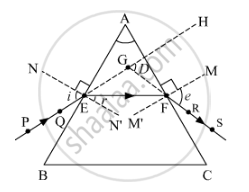Share

# The Correctly Marked Angles - CBSE Class 10 - Science

#### Question

Study the following figure in which a student has marked the angle of incidence (∠i), angle of refraction (∠r), angle of emergence (∠e), angle of prism (∠A) and the angle of deviation (∠D). The correctly marked angles are(a) ∠A and ∠i

(b) ∠A, ∠i and ∠r

(c) ∠A, ∠i, ∠e and ∠D

(d) ∠A, ∠i, ∠r and ∠D

#### Solution

The correctly marked angles in the given figure are the angle of prism (A) and the angle of incidence (i).

All the angles are correctly marked in the figure shown below.Hence, the correct answer is option (a).

Is there an error in this question or solution?

#### APPEARS IN

Solution The Correctly Marked Angles Concept: Dispersion by a Prism.
S